# Playing with DeFi Pulse API

in LeoFinance2 years ago

DeFi Pulse provides an API, which allows to gather data about several DeFi platforms. I decided to call the API and do some data exploration, and see what kind of insights it could provide.

``````import pandas as pd
import plotly.express as px
``````

## Request from Defi Pulse API

``````import requests
url = "https://data-api.defipulse.com/api/v1/defipulse/api/GetProjects?api-key=my_key"
api_data = requests.get(url)
``````

## Convert JSON to Data Frame

``````df = pd.DataFrame.from_dict(api_data.json())
df.to_json('defi_pulse_data.json')
``````

No need to call the API again, if you save it as a JSON file:

``````df = pd.read_json('defi_pulse_data.json')
``````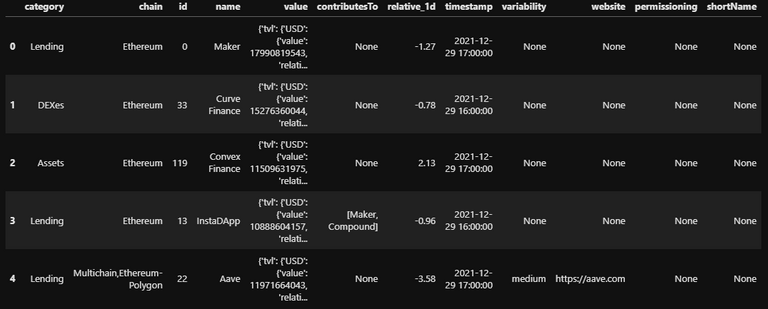Extract Total Value Locked (TVL) in USD from `value`:

``````def extract_value_usd(x):
value = x['tvl']['USD']['value']
return value

df['tvl_usd'] = df.value.apply(extract_value_usd)

df = df[['id', 'name', 'chain', 'category', 'tvl_usd', 'timestamp']]
``````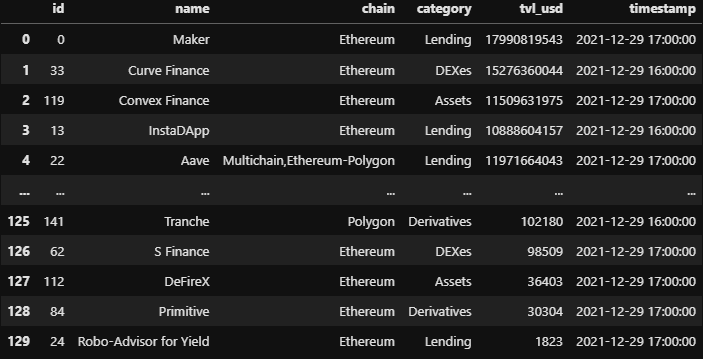## Exploratory Data Analysis

``````df = df.sort_values(by='id', ascending=True)
df.chain = df.chain.apply(lambda x: x.capitalize())
df.chain.value_counts()
``````
``````Ethereum                       122
Polygon                          4
Multichain,ethereum-polygon      3
Bitcoin                          1
Name: chain, dtype: int64
``````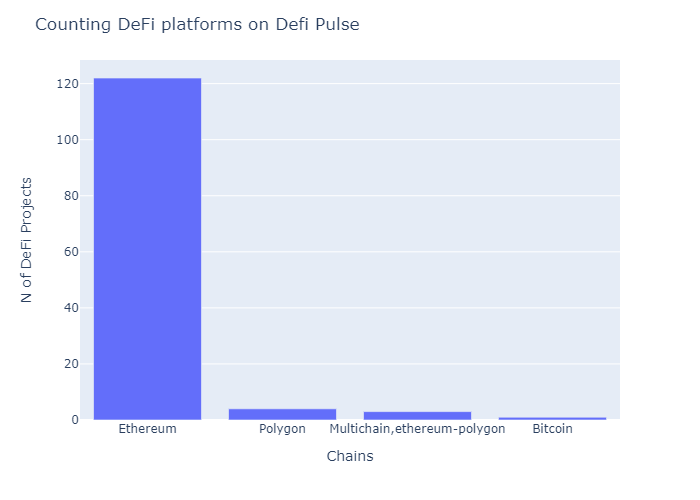Ethereum is clearly the chain with the most DeFi projects (according to DefiPulse).

``````df[['chain', 'tvl_usd']].groupby('chain').sum('tvl_usd') \
.sort_values(by='tvl_usd', ascending = False)
``````
CHAINTVL_USD
Ethereum104215823028
Multichain,ethereum-polygon12117665841
Bitcoin159153053
Polygon87082896

Also the Blockchain with the most Total Value Locked on DeFi.

## Getting DeFi platforms with the most and the least TVL

To get the platforms with the most TVL, I observed the ones above the `75th quantile`.

``````df_Q75 = df.loc[df.tvl_usd > df.describe() \
.reset_index().tvl_usd].sort_values(by='tvl_usd', ascending=False)

df_Q25 = df.loc[df.tvl_usd < df.describe() \
.reset_index().tvl_usd].sort_values(by='tvl_usd', ascending=False)
``````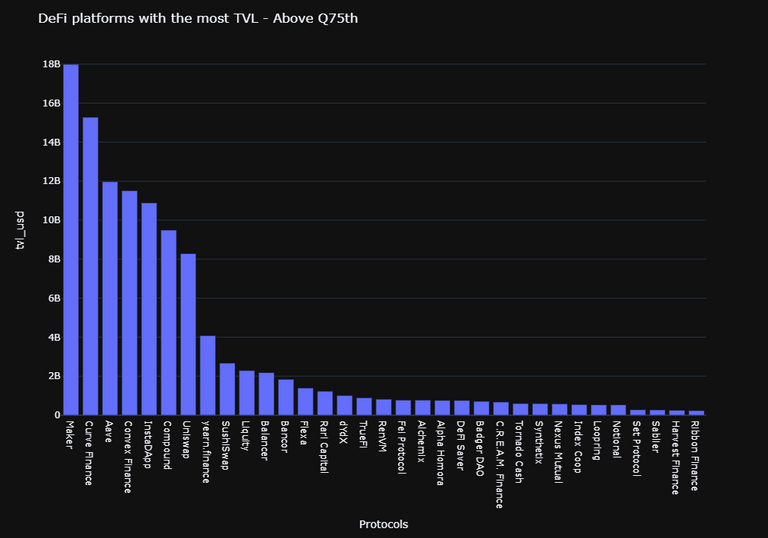To get the platforms with the least TVL, I observed the ones below the `25th quantile`.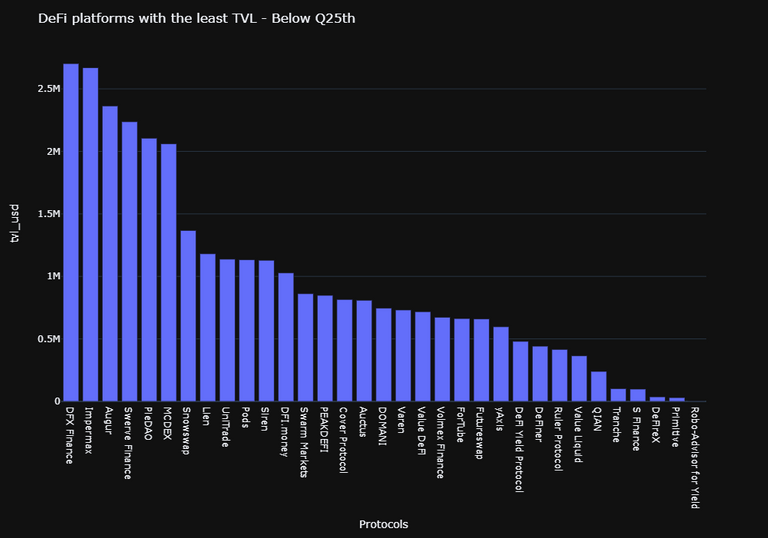## Categories

The TVL is locked in different categories, such as: `Lending`, `DEXes`, `Assets`, `Derivates`, `Payments`. In the barplot below we can see how the value is dispersed in these 5 categories.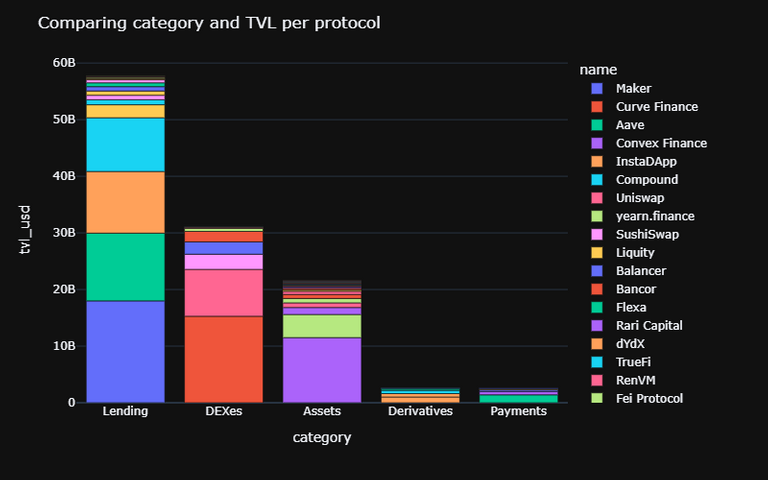Below, the barplot compares the TVL and the category per chain. Both Ethereum and Multichain are hidden, in order to better visualize both Bitcoin and Polygon.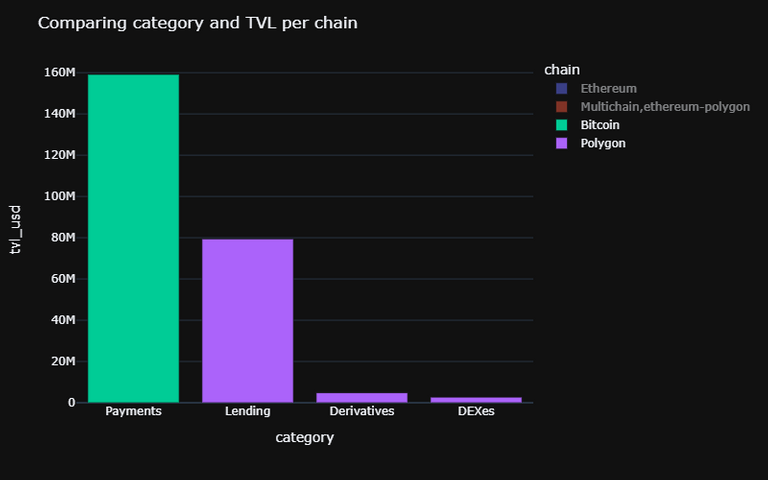In the case of Bitcoin, the TVL from the Payments category comes from the Lightning Network.

In addition, 58% of DeFi's wealth is locked in only 5 platforms: `Maker`, `Curve Finance`, `Aave`, `Convex Finance` and `InstaDapp`.

## Future Exploration

It would be interesting to analyse more deeply the platforms with the most TVL and get insights about their success, much probably using data from another source. On the other side, the platforms with the least TVL should be better studied, to see if they can be a good investment or, for instance, if they provide a good APR/APY.